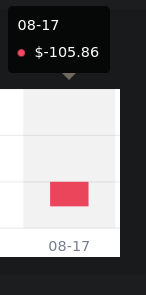Hello,

# Introduction of the problem

For the need of a professional project, i have to reproduce the exact calculation (~\$1 variation) from Binance “Spot Profit & Loss Analysis” page.

`Daily PNL = Daily final asset in spot account - Initial asset at 00:00:00 UTC - Net transfer and deposit`

But no matter what I try, I’m unable to obtain the values given on the Binance website P&L with the API.

# Demonstration

I have assets in:

• BTC
• ETH
• BNB
• USDT

• BTC/USDT
• ETH/USDTa
• BNB/USDT

For a day with no order/trade, no deposit and no withdraw, my program give me the following datas:
I included the timestamp that can be verified here: epochconverter. com

``````INFO At 2021-08-17 00:00:00 [TS: 1629158400000], for BTC / USDT price was 45901.30000000 and volume was 0.0429570000
INFO At 2021-08-17 00:00:00 [TS: 1629158400000], for ETH / USDT price was 3147.18000000 and volume was 0.0021600000
INFO At 2021-08-17 00:00:00 [TS: 1629158400000], for BNB / USDT price was 415.72000000 and volume was 3.1028793300
INFO USDT / USDT: Volume was 1421.2190474600

INFO At 2021-08-17 23:59:59.999000 [TS: 1629244799999], for BTC / USDT price was 44695.95000000 and volume was 0.0429570000
INFO At 2021-08-17 23:59:59.999000 [TS: 1629244799999], for ETH / USDT price was 3011.72000000 and volume was 0.0021600000
INFO At 2021-08-17 23:59:59.999000 [TS: 1629244799999], for BNB / USDT price was 401.80000000 and volume was 3.1028793300
INFO USDT / USDT: Volume was 1421.2190474600
``````

So far, we have this values.

Open value BTC/USDT: `45901.30000000 * 0.0429570000`
Open value ETH/USDT: `3147.18000000 * 0.0021600000`
Open value BNB/USDT: `415.72000000 * 3.1028793300`
Open value USDT/USDT: `1.000* 1421.2190474600`

Close value BTC/USDT: `44695.95000000 * 0.0429570000`
Close value ETH/USDT: `3011.72000000 * 0.0021600000`
Close value BNB/USDT: `401.80000000 * 3.1028793300`
Close value USDT/USDT: `1.000* 1421.2190474600`

So when I try to do a manual calculation:

P&L = `Close value Assets - Open Value Assets`

P&L = `( Close value BTC/USDT + Close value ETH/USDT + Close value BNB/USDT + Close value USDT/USDT) - (Open value BTC/USDT + Open value ETH/USDT + Open value BNB/USDT + Open value USDT/USDT)`

P&L =
`((44695.95 * 0.042957)+(3011.72 * 0.00216)+(401.80 * 3.10287933)+(1.000* 1421.21904746)) - ((45901.30 * 0.042957)+(3147.180 * 0.002160)+(415.720 * 3.102879330)+(1.000* 1421.2190474600))`

P&L= −95.262893824

And here is the result from binance:Today is the same:

• -19.6046472603\$ for my calculation.
• -11.94\$ for Binance.

# Conclusion

I’ve tried everything, but no matter what parameter I tried to manage, I have ALWAYS a 5-10% difference.
I know I must have missed something, but an employee from Binance could not resolve my problem too when discussed on telegram.

Why there is no route to get daily P&L ?

Anyone ever achieved this ? I seems to be the only one struggling (or interested) with this.

Or someone at Binance could check my Data if they are correct ?

# Code

I have a program in python with the python Binance library python-binance

Here is the flow:

• At 00:01:00 UTC everyday, I save my account balance in Database.
• When I need to calculate P&L:
• First for each Pair
• I use the route `/api/v3/klines` with 1 day as interval to retrieve the price of my asset (I.E: BTC/USDT at 00:00:00 UTC and 23:59:99 99999 UTC)
• I get one I use the 2nd and 5th field (index 1 and 4) of the single kline that Binance APi send back.
• I calculate the difference between my assets values
• Then I sums the differences for each pair.

My function to calculate P&L for the day

``````    # Retrieving open and close balances in Database
try:
open_balance = balances.get(date=start.date())
close_balance = balances.get(date=end.date())
except Exception as e:
raise e

# Retrieving open and close price
# https://binance-docs.github.io/apidocs/spot/en/#kline-candlestick-data
opening_price = Decimal(kline)
closing_price = Decimal(kline)

# Computing open and close assets values with assets balance volumes at open and close time
open_value = opening_price * open_balance.base_volume
close_value = closing_price * close_balance.base_volume

return {
'Open': opening_price,
'Close': closing_price,
'PriceDiff': float(closing_price - opening_price),
'OpenValue': float(open_value),
'CloseValue': float(close_value),
'ValueDiff': float(close_value - open_value)
}

``````

My loop for P&L for each day (even if it’s not necessary with the example above).

``````    day = start_date
day_after = start_date + timedelta(days=1)
i = 0
pair_pnl = {}

try:
while day_after <= end_date:
# Retrieving daily values for current pair
pair_daily_pln = pair_pnl_for_day(balances, klines[i], day, day_after, euro)
if pair_daily_pln is None:
raise "calculate_pair_pnl: daily_pnl_for_pair returned None"

# Adding daily values to the dict with date as key
pair_pnl[day.strftime('%Y-%m-%d')] = pair_daily_pln

# Moving one day forward
i += 1
day, day_after = day_after, day_after + timedelta(days=1)
except Exception as e:
print(f"When dealing with pair {pair.symbol}: {e}", e)
raise e
return pair_pnl
``````

I also need this. I hope binance official reply to this